# Concrete box

The concrete box with walls thick 7 cm has the following external dimensions: length 1.8 m, width 44 cm and height 46 cm. How many liters of soil can fit if I fill it to the brim?

x =  194.2 l

### Step-by-step explanation: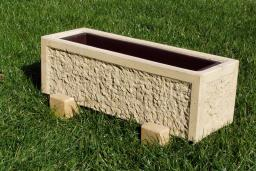Did you find an error or inaccuracy? Feel free to write us. Thank you!Tips to related online calculators
Do you want to convert length units?
Do you know the volume and unit volume, and want to convert volume units?

## Related math problems and questions:

• FlowerpotThe block-shaped flowerpot has external dimensions: length 1.25 m, width 10 cm, and height 11 cm. The thickness of the boards from which it is made is 0.8 cm. How many liters of soil is needed to fill it 1 cm below the top edge? What surface do we have to
• The cuboidThe cuboid is full to the brim with water. The external dimensions are 95 cm, 120 cm, and 60 cm. The thickness of all walls and the bottom is 5 cm. How many liters of water fit into the cuboid?
• Milk packageMilk is sold in a box with dimensions of 9.5 cm; 16.5 cm and 6.5 cm. Determine the maximum amount of milk that can fit into a box. Coating thickness is negligible.
• Base of houseCalculate the volume of the bases of a square house, if the base depth is 1.2 m, the width is 40 cm and their outer circumference is 40.7 m.
• Concrete tank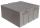How many m3 of concrete is in the tank shape of a cuboid with dimensions of 2000 cm and 5.6 meters and 70 dm if the concrete takes up 3/7 of the tank?
• Stone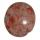When Peter threw stone in a box of water he discovered that the water level has risen by 6 cm. The box has a cuboid shape, the bottom has dimensions of 24 cm and 14 cm, height is 40 cm. What volume has a stone?
• ConvaHow many liters of water fit into the shape of a cylinder with a bottom diameter 20 cm and a height 45 cm?
• Edges of the cuboid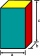Find the length of the edges of the cuboid, which has the following dimensions: width is 0.4 m; the height is 5.8 dm and the block can hold 81.2 liters of fluid.
• Cuboid boxHow many m2 paper is needed for the sticking cuboid box of dimensions 50 cm, 40 cm and 30 cm? To the folds add one-tenth the area.
• Pool in litresPool has a width of 3.5 m length of 6 m and a height 1.60 meters. Calculate pool volume in liters.
• PoolMr. Peter builds a pool shape of a four-sided prism with a rhombus base in the garden. Base edge length is 8 m, the distance of the opposite walls of the pool is 7 m. The estimated depth is 144 cm. How many hectoliters of water consume Mr. Peter to fill t
• FreezerThe freezer has the shape of a cuboid with internal dimensions of 12 cm, 10 cm, 30 cm. A layer of ice of 23 mm thick was formed on the inner walls (and on the opening) of the freezer. How many liters of water will drain if we dispose the freezer?
• AquariumAquarium is rectangular box with square base containing 76 liters of water. Length of base edge is 42 cm. To what height the water level goes?
• Aquarium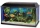I have an aquarium that is 100 cm long and 40 cm wide and 40 cm in height. We fill it with water. How much will it weigh?
• Pool 3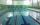How long will fill pool cuboid shape (8m 6m 1.5m) when flows 15 liters/s?
• Truck of milk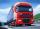How many hectoliters of ,,the box" milk fit in the truck, ake cargo size area is 2.8 m x 3 m x 17 m? A liter of milk in a box measuring 12 cm x 7 cm x 20 cm.
• The wall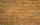We have to build a cuboid wall with dimensions base 30 cm and 45 cm and height 3.25 meters. Calculate how many we need bricks if we spend 400 pieces of bricks to 1 m3 of wall?